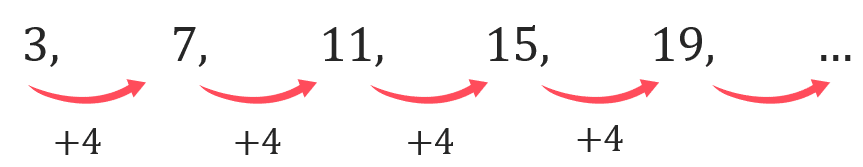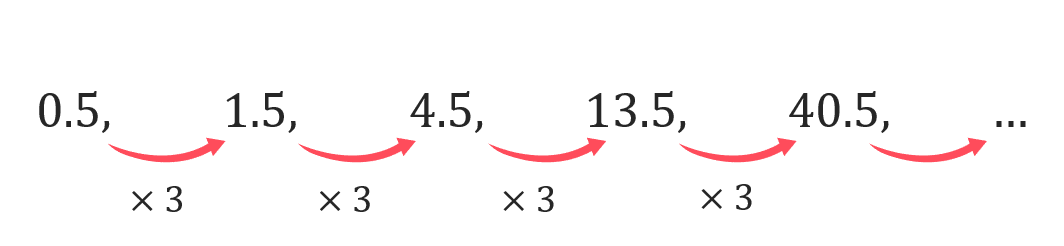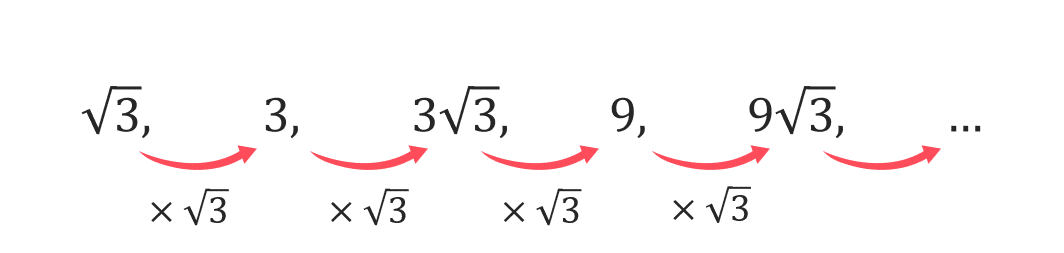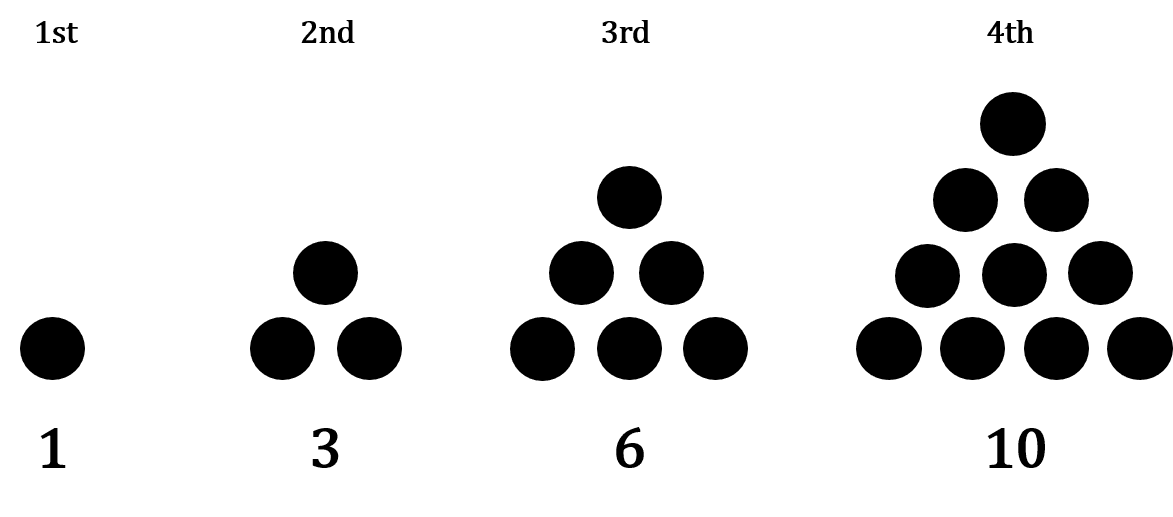# Sequences and Nth Term

GCSEKS3Level 4-5Level 6-7AQACambridge iGCSEEdexcelEdexcel iGCSEOCRWJEC

## Sequences

In maths, a sequence is a list of numbers, algebraic terms, shapes, or other mathematical objects that follow a pattern or rule. There are two different ways you will be expected to work out a sequence:

• A term-to-term rule – each term in the sequence is calculated by performing a fixed set of operations (such as “multiply by $2$ and add $3$”) to the term(s) before it.
• A position-to-term rule – each term in the sequence is calculated according to its position in the sequence. Usually, this takes the form of an $n^{th}$ term formula.

Level 4-5GCSEKS3AQAEdexcelOCRWJECCambridge iGCSEEdexcel iGCSE

## Finding a Sequence from the $n^{th}$ Term

Some sequences have a rule called an $n^{th}$ term rule, which tells you how to generate terms.

The $n^{th}$ term rule is always an expression in $n$.

To find the first term in the sequence, substitute $n=1$ into the expression. To find the second term in the sequence, substitute $n=2$ into the expression. And so on.

Example: A sequence has the $n^{th}$ term rule $2n+1$. Find the first four terms.

First term: $n=1$

$2n+1=2\times1+1=3$

Second term: $n=2$

$2n+1=2\times2+1=5$

Third term: $n=3$

$2n+1=2\times3+1=7$

Fourth term: $n=4$

$2n+1=2\times4+1=9$

The first four terms are: $3$, $5$, $7$ and $9$.

Level 4-5GCSEKS3AQAEdexcelOCRWJECCambridge iGCSEEdexcel iGCSE
Level 4-5GCSEKS3AQAEdexcelOCRWJECCambridge iGCSEEdexcel iGCSE

## Finding the $n^{th}$ term for a Linear Sequence

Linear sequences (or arithmetic progressions) are sequences that increase or decrease by the same amount between each term.

Want a way to express any term in a concise mathematical way? This can be done using the $n^{th}$ term formula. This is a rule that gives you the value of any term in the sequence in the form,

$an+b,$

Where $a$ and $b$ are numbers to be determined.

Example: Find the $n^{th}$ term for the following sequence,    $3, \, 7, \,11, \,15, \,19, \, ...$Step 1: Find the Common Difference ($a$)

The common difference is the amount the sequence increases (or decreases) each time.

$a=4$, because $a$ is always the difference between each term.

Step 2: Determine if you need to Add or Subtract anything ($b$)

To work out $b$, consider the sequence formed by putting $n=1, 2, 3, 4, 5$ into $4n$:

$4, 8, 12, 16, 20$

What’s the difference between these terms and our actual sequence? They’re all too big by $1$. So, to make our original sequence, we must subtract $1$ from $4n$.

Step 3: Write the formula in the correct form ($an+b$)

Thus, our $n^{th}$ term formula is

$4n-1$.

Level 4-5GCSEKS3AQAEdexcelOCRWJECCambridge iGCSEEdexcel iGCSE

## Geometric Sequences

Another type of sequence is a geometric sequence or geometric progression.

In a geometric sequence, you multiply each term by a common ratio to get to the next term. For example,is a geometric progression where to get get to the next term you have to multiply the previous term by the common ratio. Therefore to find the next two terms of this sequence we have to multiply the preceding term by $3$, so

$40.5\times 3=121.5$ and  $121.5\times 3 =364.5$

Level 4-5GCSEKS3AQAEdexcelOCRWJECCambridge iGCSEEdexcel iGCSE
Level 6-7GCSEAQAEdexcelOCRCambridge iGCSEEdexcel iGCSE

## Geometric Sequences involving Surdsis a geometric progression where the common ratio is not a rational number. The method of finding the next two terms of this sequence is the same as before, multiply the preceding term by $\sqrt{3}$, so

$9\sqrt{3}\times 3=27$ and  $27\times \sqrt{3} = 27\sqrt{3}$

Level 6-7GCSEAQAEdexcelOCRCambridge iGCSEEdexcel iGCSE

## Other Types of Sequences

There are other types of sequences you should be familiar with:

## Triangular Numbers

Triangular numbers are numbers that can be represented as an equilateral triangle of dots.The $n^{th}$ term is $\dfrac{n(n+1)}{2}$, giving

$1, 3, 6, 10, 15, 21, ...$

## Square Numbers

These sequences are made up of square numbers so the $n^{th}$ term is $n^2$, giving

$1, 4, 9, 16, 25, 36, 49, ...$

## Cubic Numbers

These sequences are made up of cubic numbers so the $n^{th}$ term is $n^3$, giving

$1, 8, 27, 64, 125, 216, ...$

## The Fibonacci Numbers

The first few terms of the Fibonacci sequence are:

$1, 1, 2, 3, 5, 8, 13, 21, ...$

This is a famous sequence that you need to recognise. The rule is the previous $2$ terms are added together in the sequence to get the next term.

Level 4-5GCSEKS3AQAEdexcelOCRWJECCambridge iGCSEEdexcel iGCSE

## Note:

Subscript notation can be used to denote position to term and term to term rules.

For example

$x_{n+1}=3x_n-4$ shows that you multiply the previous term by $3$ and subtract $4$ to get the next term.

$y_n=5n+3$ gives you a rule for the $n$th term of a sequence.

Level 4-5GCSEOCR
Level 4-5GCSEKS3AQAEdexcelOCRWJECCambridge iGCSEEdexcel iGCSE

## Example 1: Linear Sequences

Find the $n^{th}$ term formula for the sequence $-2, 5, 12, 19, 26$.

[2 marks]

The first step is to find the common difference between each term.Hence we can write the $n^{th}$ term as,

$7n+b$

To work out $b$, consider the sequence formed by putting $n=1, 2, 3, 4, 5$ into $u_n=7n$:

$7, 14, 21, 28, 35$

The difference between these numbers and our sequence is we need to subtract $9$ from each term. Thus, our $n^{th}$ term is

$7n-9$.

Level 4-5GCSEKS3AQAEdexcelOCRWJECCambridge iGCSEEdexcel iGCSE

## Example 2: Determining if a Value is Part of a Sequence

The first five terms of a sequence are:

$-3, 1, 5, 9, 13$

Determine if $1143$ is part of this sequence.

[3 marks]Step 1: First we must find the $n^{th}$ term of the sequence of the sequence as before, this gives

$4n-7$.

Step 2: Next we need to write the $n^{th}$ term as an euqation equal to $1143$ and solve for $n$

If $n$ solves to give an integer, then $1143$ is part of the sequence.

\begin{aligned} 4n-7&=1143 \\ 4n & = 1150 \\ n&= 287.5\end{aligned}

As $287.5$ is not an integer, $1143$ must not be part of the sequence.

Level 4-5GCSEKS3AQAEdexcelOCRWJECCambridge iGCSEEdexcel iGCSE

## Example 3: Solving Problems involving Sequences

The sum of two consecutive terms in a sequence given by the $n^{th}$ term, $3n+8$ is $109$. Find the values of these two terms.

[4 marks]

In this case we have to first set up an equation, setting the first term as $n$ and the second as $n+1$, such that,

\begin{aligned} 3n+8+3(n+1)+8 & = 109 \\ 3n + 8 + 3n + 3 + 8 & = 109 \\ 6n +19 &= 109 \\ 6n &= 90 \\ n &=15 \end{aligned}

Hence, it is the $15$th and $16$th terms we are looking for which are,

$3(15)+8 = 53$ and $3(16) +8 = 56$

Level 4-5GCSEKS3AQAEdexcelOCRCambridge iGCSEEdexcel iGCSE

## Sequences and Nth Term Example Questions

a) To find the $12^{th}$ term of this sequence, we will substitute $n=12$ into the formula given.

$4(12)+1=49$

So, the $12^{th}$ term is $49$

b) Every term in this sequence is generated when an integer value of $n$ is substituted into $4n+1$

Hence if we set $77$ to equal $4n+1$, we can determine its position in the sequence,

$4n+1=77$

making $n$ the subject by subtracting $1$ then dividing by $4$,

$n=\dfrac{77-1}{4}=19$

Hence $77$ is the $19^{th}$ term in the sequence.Gold Standard Education

a) To generate the first $5$ terms of this sequence, we will substitute $n=1, 2, 3, 4, 5$ into the formula given.

\begin{aligned}1 &=5(1)-4 =1 \\ 2 &=5(2)-4 =6 \\ 3 & =5(3)-4=11 \\ 4 &=5(4)-4=16 \\ 5 &=5(5)-4=21 \end{aligned}

So, the first $5$ terms are $1$, $6$, $11$, $16$, and $21$

b) Every term in this sequence is generated when an integer value of $n$ is substituted into $5n-4$.

If we set $108$ to equal $5n-4$, we can determine if it is a part of the sequence or not. If the value of $n$ is a whole number then it is part of the sequence, hence

$5n-4=108$

making $n$ the subject by adding $4$ then dividing by $5$,

$n=\dfrac{112}{5}=22.4$

As there is no “$22.4^{th}$” position in the sequence, it must the case that $108$ is not a term in this sequence.Gold Standard Education

We are told it is an arithmetic progression and so must have $n^{th}$ formula: $an+b$. To find $a$, we must inspect the difference between each term which is $5$, hence $a=5$.

Then, to find $b$, let’s consider the sequence generated by $5n$:

$5,\,\,10,\,\,15,\,\,20,\,\,25$

Every term is this sequence is bigger than the corresponding terms in the original sequence by $8$. So, to get to the original sequence, we will have to subtract $8$ from every term in this sequence. In other words, the $n^{th}$ term formula for our sequence in question is

$5n-8$Gold Standard Education

## Sequences and Nth Term Worksheet and Example Questions

### (NEW) Sequences and Nth Term (Linear) Exam Style Questions - MME

Level 4-5GCSENewOfficial MME

## Sequences and Nth Term Drill Questions

Level 4-5GCSE

### Sequences & nth term - Drill Questions

Level 4-5GCSEGold Standard Education